# 一起来写个简单的解释器（14）嵌套作用域和源到源编译器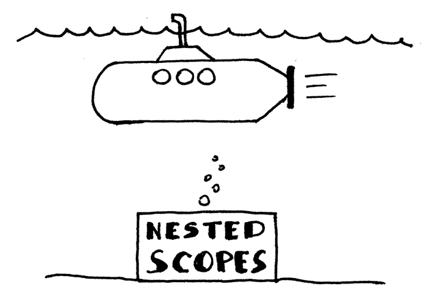• 将学习作用域，为什么它们很有用，以及如何在符号表的代码中实现它们。
• 将学习嵌套作用域以及如何使用链式作用域符号表来实现嵌套作用域。
• 将学习如何解析有形式参数的过程声明，以及如何在代码中表示过程符号。
• 将学习如何扩展我们的语义分析器，以在存在嵌套作用域的情况下进行语义检查。
• 将学习更多关于名称解析的知识 ，以及当程序有嵌套作用域时，语义分析器如何将名称解析到它们的声明。
• 将学习如何构建作用域树 。
• 今天也将学习如何编写我们自己的 源到源编译器 ！ 我们将在后面的文章中看到它与作用域讨论的相关性。

#### 作用域和作用域的符号表

``````program Main;
var x, y: integer;
begin
x := x + y;
end.
``````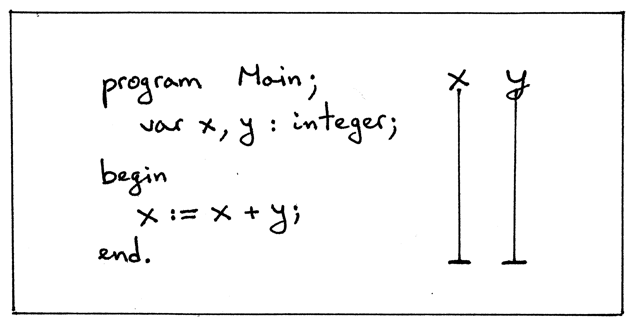Pascal程序被认为是 词法作用域 的 （或 静态作用域 ），因为甚至不需要执行程序，可以通过查看源代码，完全基于名称（引用）的文本规则来决定解析或引用哪个声明。 例如，在Pascal中，像 programend 这样的词汇关键词划分了作用域的文本边界：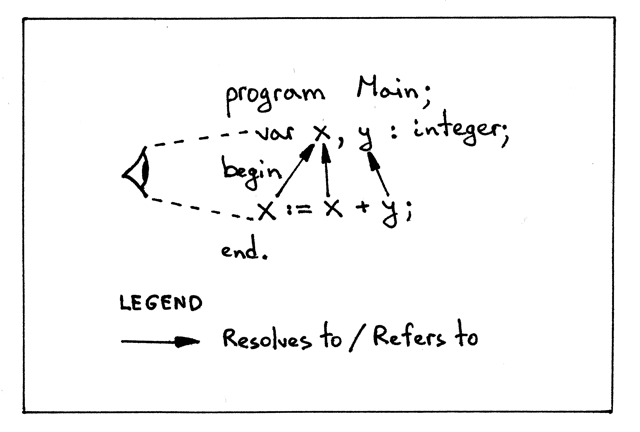• 每个作用域都会创建一个独立的名称空间，这意味着在作用域中声明的变量不能从外部访问。
• 您可以在不同的作用域内重复使用相同的名称，只需查看程序源代码即可清楚的知道，在程序中每个位置该名称所引用的具体变量声明。
• 在嵌套的作用域中，可以重新声明一个与外部作用域相同名称的变量，有效地隐藏外部声明，从而可以控制对外部作用域的不同变量的访问。

1. 每个变量（符号）在什么级别被声明
2. 名称引用的哪个声明和哪个级别的变量：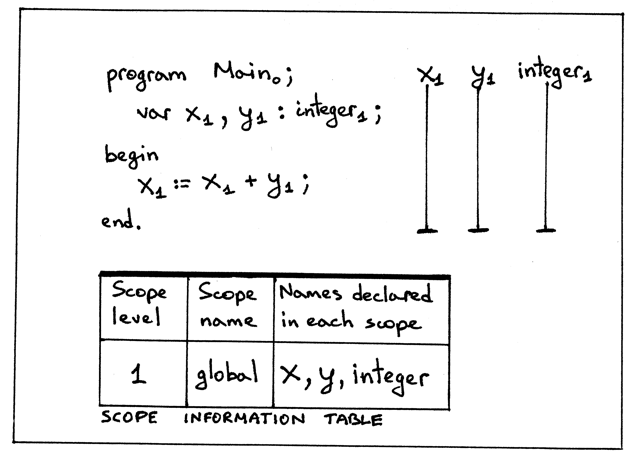• 我们有一个单一的作用域：全局作用域 ，由PROGRAM关键字引入
• 全局作用域在级别1级
• 变量（符号） x和y在第1级声明（ 全局作用域 ）。
• 内建整型类型也在第1级声明
• 程序名称Main有一个下标0。为什么程序的名字在0级，你可能想知道？ 这是为了清楚说明程序的名称不在全局作用域内 ，而在其他外部作用域内，它的级别为零。
• 变量x和y的作用域是整个程序，如垂直线所示
• 作用域信息表为程序中的每个级别显示相应的作用域级别、作用域名称和在作用域中声明的名称。 该表的目的是总结和直观地显示程序中作用域的不同信息。

``````class ScopedSymbolTable(object):
def __init__(self, scope_name, scope_level):
self._symbols = OrderedDict()
self.scope_name = scope_name
self.scope_level = scope_level
self._init_builtins()

def _init_builtins(self):
self.insert(BuiltinTypeSymbol('INTEGER'))
self.insert(BuiltinTypeSymbol('REAL'))

def __str__(self):
h1 = 'SCOPE (SCOPED SYMBOL TABLE)'
lines = ['\n', h1, '=' * len(h1)]
('Scope name', self.scope_name),
('Scope level', self.scope_level),
):
h2 = 'Scope (Scoped symbol table) contents'
lines.extend([h2, '-' * len(h2)])
lines.extend(
('%7s: %r' % (key, value))
for key, value in self._symbols.items()
)
lines.append('\n')
s = '\n'.join(lines)
return s

__repr__ = __str__

def insert(self, symbol):
print('Insert: %s' % symbol.name)
self._symbols[symbol.name] = symbol

def lookup(self, name):
print('Lookup: %s' % name)
symbol = self._symbols.get(name)
# 'symbol' is either an instance of the Symbol class or None
return symbol
``````

``````class SemanticAnalyzer(NodeVisitor):
def __init__(self):
self.scope = ScopedSymbolTable(scope_name='global', scope_level=1)

...
``````

``````\$ python scope01.py
Insert: INTEGER
Insert: REAL
Lookup: INTEGER
Insert: x
Lookup: INTEGER
Insert: y
Lookup: x
Lookup: y
Lookup: x

SCOPE (SCOPED SYMBOL TABLE)
===========================
Scope name     : global
Scope level    : 1
Scope (Scoped symbol table) contents
------------------------------------
INTEGER: <BuiltinTypeSymbol(name='INTEGER')>
REAL: <BuiltinTypeSymbol(name='REAL')>
x: <VarSymbol(name='x', type='INTEGER')>
y: <VarSymbol(name='y', type='INTEGER')>
``````

#### 具有形式参数的过程的声明

``````program Main;
var x, y: real;

procedure Alpha(a : integer);
var y : integer;
begin
x := a + x + y;
end;

begin { Main }

end.  { Main }
``````

``````ASIDE ： 形式参数是在程序声明中显示的参数。 参数 （也称为实际参数 ）是在特定过程调用中传递给过程的不同变量和表达式。
``````

1. 添加 Param AST节点

``````class Param(AST):
def __init__(self, var_node, type_node):
self.var_node = var_node
self.type_node = type_node
``````
2. 更新 ProcedureDecl 节点的构造函数以获取参数： params

``````class ProcedureDecl(AST):
def __init__(self, proc_name, params, block_node):
self.proc_name = proc_name
self.params = params  # a list of Param nodes
self.block_node = block_node
``````
3. 更新 declarations 规则以反映过程声明子规则中的更改

``````def declarations(self):
"""declarations : (VAR (variable_declaration SEMI)+)*
| (PROCEDURE ID (LPAREN formal_parameter_list RPAREN)? SEMI block SEMI)*
| empty
"""
``````
4. 添加 formal_parameter_list 规则和方法

``````def formal_parameter_list(self):
""" formal_parameter_list : formal_parameters
| formal_parameters SEMI formal_parameter_list
"""
``````
5. 添加 formal_parameters 规则和方法

``````def formal_parameters(self):
""" formal_parameters : ID (COMMA ID)* COLON type_spec """
param_nodes = []
``````

``````procedure Foo;

procedure Foo(a : INTEGER);

procedure Foo(a, b : INTEGER);

procedure Foo(a, b : INTEGER; c : REAL);
``````

``````\$ python genastdot.py nestedscopes02.pas > ast.dot && dot -Tpng -o ast.png ast.dot
``````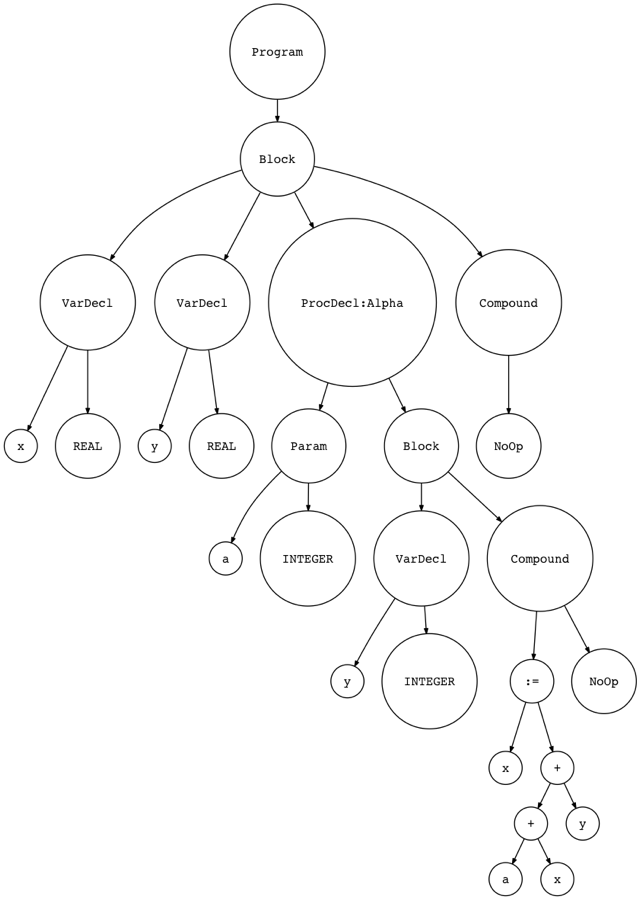#### 过程符号

``````class ProcedureSymbol(Symbol):
def __init__(self, name, params=None):
super(ProcedureSymbol, self).__init__(name)
# a list of formal parameters
self.params = params if params is not None else []

def __str__(self):
return '<{class_name}(name={name}, parameters={params})>'.format(
class_name=self.__class__.__name__,
name=self.name,
params=self.params,
)

__repr__ = __str__
``````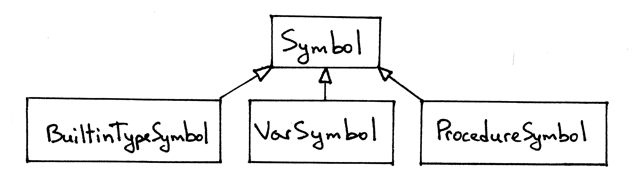#### 嵌套作用域

``````program Main;
var x, y: real;

procedure Alpha(a : integer);
var y : integer;
begin
x := a + x + y;
end;

begin { Main }

end.  { Main }
``````• 这个Pascal程序有两个作用域级别：1级和2级
• 嵌套关系图可视化地显示作用域 Alpha 嵌套在全局作用域内 ，因此有两个级别： 在级别1的全局作用域和在级别2的 Alpha 作用域。
• 过程声明 Alpha 的作用域级别比在过程 Alpha 中声明的变量级别小1。 您可以看到，过程声明 Alpha 的作用域级别是1，过程中变量a和y的作用域级别是2。
• Alpha 过程中y的变量声明隐藏了y在全局作用域内的声明。 你可以在y1的垂直栏中看到这个洞（顺便说一下，1是下标，它不是变量名的一部分，变量名只是y ），你可以看到y2变量声明的作用域是 Alpha 过程的整个身体。
• 您已经知道，作用域信息表显示了作用域级别，这些级别的作用域名称以及这些作用域（在这些级别）中分别声明的相应名称。
• 在图中，也可以看到，我省略了整数和实数类型的作用域（除了在作用域信息表中），因为它们总是在作用域级别1（ 全局作用域）中声明 ，所以我不会对整型和实型加下标来节省视觉空间，但是在表示全局作用域的作用域符号表的内容中会一次又一次地看到这些类型。

``````program Main;
var x, y: real;

procedure Alpha(a : integer);
var y : integer;
begin

end;

begin { Main }

end.  { Main }
``````

``````def visit_Program(self, node):
print('ENTER scope: global')
global_scope = ScopedSymbolTable(
scope_name='global',
scope_level=1,
)
self.current_scope = global_scope

# visit subtree
self.visit(node.block)

print(global_scope)
print('LEAVE scope: global')
``````

1. 当访问AST中的节点时，我们首先打印进入的作用域，在这个例子中是 全局
2. 我们创建一个单独的作用域符号表来表示全局作用域 。 当构造一个 ScopedSymbolTable 的实例时，我们明确地将作用域名称和作用域级别参数传递给类构造函数。
3. 我们将新创建的作用域分配给实例变量 current_scope 。 在作用域化符号表中插入和查找符号的其他访问者方法将使用 current_scope
4. 我们访问一个子树（块）。 这是旧的部分。
5. 在离开全局作用域之前，我们打印全局作用域的内容（作用域符号表）
6. 我们还打印出我们正在离开全局作用域的信息

``````def visit_ProcedureDecl(self, node):
proc_name = node.proc_name
proc_symbol = ProcedureSymbol(proc_name)
self.current_scope.insert(proc_symbol)

print('ENTER scope: %s' %  proc_name)
# Scope for parameters and local variables
procedure_scope = ScopedSymbolTable(
scope_name=proc_name,
scope_level=2,
)
self.current_scope = procedure_scope

# Insert parameters into the procedure scope
for param in node.params:
param_type = self.current_scope.lookup(param.type_node.value)
param_name = param.var_node.value
var_symbol = VarSymbol(param_name, param_type)
self.current_scope.insert(var_symbol)
proc_symbol.params.append(var_symbol)

self.visit(node.block_node)

print(procedure_scope)
print('LEAVE scope: %s' %  proc_name)
``````

1. 该方法所做的第一件事就是创建一个过程符号并将其插入到当前作用域中，这是我们示例程序的全局作用域 。
2. 然后该方法打印有关进入过程作用域的消息。
3. 然后我们为过程的参数和变量声明创建一个新的作用域。
4. 我们将过程作用域指定给 self.current_scope 变量，表明这是我们当前的作用域，所有符号操作（ 插入和查找 ）将使用当前作用域。
5. 然后将过程的形式参数插入当前作用域并将它们添加到过程符号中
6. 然后我们访问AST子树的其余部分——过程的主体。
7. 最后，我们在离开节点之前打印关于离开作用域的消息，并移动到另一个AST节点（如果有的话）。

``````def visit_VarDecl(self, node):
type_name = node.type_node.value
type_symbol = self.current_scope.lookup(type_name)

# We have all the information we need to create a variable symbol.
# Create the symbol and insert it into the symbol table.
var_name = node.var_node.value
var_symbol = VarSymbol(var_name, type_symbol)

self.current_scope.insert(var_symbol)

def visit_Var(self, node):
var_name = node.value
var_symbol = self.current_scope.lookup(var_name)
if var_symbol is None:
raise Exception(
)
``````

visit_VarDeclvisit_Var 现在都将使用 current_scope 来插入和/或查找符号。 具体而言，对于我们的示例程序， current_scope 可以指向全局作用域或 Alpha 作用域。

``````class SemanticAnalyzer(NodeVisitor):
def __init__(self):
self.current_scope = None
``````

``````\$ python scope02.py
ENTER scope: global
Insert: INTEGER
Insert: REAL
Lookup: REAL
Insert: x
Lookup: REAL
Insert: y
Insert: Alpha
ENTER scope: Alpha
Insert: INTEGER
Insert: REAL
Lookup: INTEGER
Insert: a
Lookup: INTEGER
Insert: y

SCOPE (SCOPED SYMBOL TABLE)
===========================
Scope name     : Alpha
Scope level    : 2
Scope (Scoped symbol table) contents
------------------------------------
INTEGER: <BuiltinTypeSymbol(name='INTEGER')>
REAL: <BuiltinTypeSymbol(name='REAL')>
a: <VarSymbol(name='a', type='INTEGER')>
y: <VarSymbol(name='y', type='INTEGER')>

LEAVE scope: Alpha

SCOPE (SCOPED SYMBOL TABLE)
===========================
Scope name     : global
Scope level    : 1
Scope (Scoped symbol table) contents
------------------------------------
INTEGER: <BuiltinTypeSymbol(name='INTEGER')>
REAL: <BuiltinTypeSymbol(name='REAL')>
x: <VarSymbol(name='x', type='REAL')>
y: <VarSymbol(name='y', type='REAL')>
Alpha: <ProcedureSymbol(name=Alpha, parameters=[<VarSymbol(name='a', type='INTEGER')>])>

LEAVE scope: global
``````

1. 您可以看到，两行 Insert: INTEGERInsert: REAL 在输出中重复两次， INTEGERREAL 关键字在两个作用域（作用域符号表）中都存在： globalAlpha 。 原因是我们为每个作用域创建一个单独的作用域符号表，并且每次创建实例时，初始化内置类型符号。 稍后我们将在讨论嵌套关系时修改它以及如何在代码中表示它们。
2. 看到行 Insert: Alpha 是如何在行 ENTER scope: Alpha 前打印的 。 这只是提示一个过程的名字被声明在一个比在过程本身中声明的变量级别低一级的级别。
3. 您可以通过检查上面的作用域符号表的打印内容来看到它们包含哪些声明。 例如，全局作用域中有 Alpha 符号。
4. 从全局作用域的内容中，还可以看到 Alpha 过程的过程符号还包含过程的形式参数。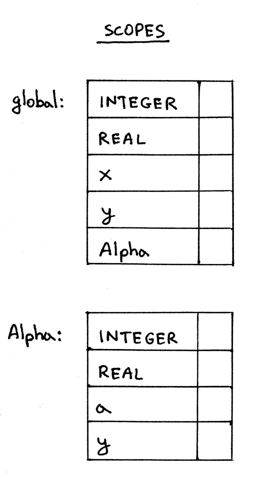#### 作用域树：链接作用域符号表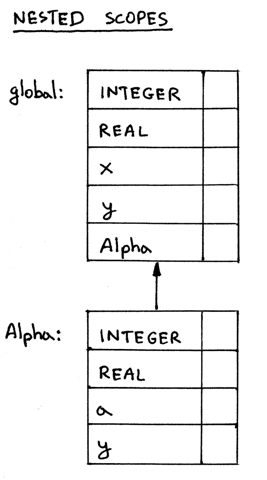1. 我们需要更新 ScopedSymbolTable 类，并添加一个变量 enclosing_scope ，该变量将包含一个指向作用域的封闭作用域的指针。这将是上图中作用域之间的链接。
2. 我们需要更新 visit_Programvisit_ProcedureDecl 方法，以使用 ScopedSymbolTable 类的更新版本创建到作用域的封闭作用域的实际链接。

``````class ScopedSymbolTable(object):
def __init__(self, scope_name, scope_level, enclosing_scope=None):
self._symbols = OrderedDict()
self.scope_name = scope_name
self.scope_level = scope_level
self.enclosing_scope = enclosing_scope
self._init_builtins()

def _init_builtins(self):
self.insert(BuiltinTypeSymbol('INTEGER'))
self.insert(BuiltinTypeSymbol('REAL'))

def __str__(self):
h1 = 'SCOPE (SCOPED SYMBOL TABLE)'
lines = ['\n', h1, '=' * len(h1)]
('Scope name', self.scope_name),
('Scope level', self.scope_level),
('Enclosing scope',
self.enclosing_scope.scope_name if self.enclosing_scope else None
)
):
h2 = 'Scope (Scoped symbol table) contents'
lines.extend([h2, '-' * len(h2)])
lines.extend(
('%7s: %r' % (key, value))
for key, value in self._symbols.items()
)
lines.append('\n')
s = '\n'.join(lines)
return s

__repr__ = __str__

def insert(self, symbol):
print('Insert: %s' % symbol.name)
self._symbols[symbol.name] = symbol

def lookup(self, name):
print('Lookup: %s' % name)
symbol = self._symbols.get(name)
# 'symbol' is either an instance of the Symbol class or None
return symbol
``````

``````def visit_Program(self, node):
print('ENTER scope: global')
global_scope = ScopedSymbolTable(
scope_name='global',
scope_level=1,
enclosing_scope=self.current_scope, # None
)
self.current_scope = global_scope

# visit subtree
self.visit(node.block)

print(global_scope)

self.current_scope = self.current_scope.enclosing_scope
print('LEAVE scope: global')
``````

1. 创建作用域时，我们显式传递 self.current_scope 作为 enclosing_scope 参数
2. 我们将新创建的全局作用域赋值给变量 self.current_scope
3. 在离开 Program 节点之前，我们将变量 self.current_scope 恢复到之前的值。 在完成处理节点之后，恢复 current_scope 的值很重要，否则当我们的程序中有两个以上的作用域时，作用域树的构造将被破坏。 我们马上就会明白为什么。

``````def visit_ProcedureDecl(self, node):
proc_name = node.proc_name
proc_symbol = ProcedureSymbol(proc_name)
self.current_scope.insert(proc_symbol)

print('ENTER scope: %s' %  proc_name)
# Scope for parameters and local variables
procedure_scope = ScopedSymbolTable(
scope_name=proc_name,
scope_level=self.current_scope.scope_level + 1,
enclosing_scope=self.current_scope
)
self.current_scope = procedure_scope

# Insert parameters into the procedure scope
for param in node.params:
param_type = self.current_scope.lookup(param.type_node.value)
param_name = param.var_node.value
var_symbol = VarSymbol(param_name, param_type)
self.current_scope.insert(var_symbol)
proc_symbol.params.append(var_symbol)

self.visit(node.block_node)

print(procedure_scope)

self.current_scope = self.current_scope.enclosing_scope
print('LEAVE scope: %s' %  proc_name)
``````

1. 创建作用域时，我们显式传递 self.current_scope 作为 enclosing_scope 参数。
2. 我们不再对过程声明的作用域级别进行硬编码，因为我们可以根据过程的封闭作用域的作用域级别自动计算出来级别：封闭作用域的级别加1。
3. 在离开 ProcedureDecl 节点之前，我们将 self.current_scope 的值恢复为之前的值（对于我们的示例程序，以前的值将是全局作用域 ）。

``````program Main;
var x, y: real;

procedure Alpha(a : integer);
var y : integer;
begin

end;

begin { Main }

end.  { Main }
``````

``````\$ python scope03a.py
ENTER scope: global
Insert: INTEGER
Insert: REAL
Lookup: REAL
Insert: x
Lookup: REAL
Insert: y
Insert: Alpha
ENTER scope: Alpha
Insert: INTEGER
Insert: REAL
Lookup: INTEGER
Insert: a
Lookup: INTEGER
Insert: y

SCOPE (SCOPED SYMBOL TABLE)
===========================
Scope name     : Alpha
Scope level    : 2
Enclosing scope: global
Scope (Scoped symbol table) contents
------------------------------------
INTEGER: <BuiltinTypeSymbol(name='INTEGER')>
REAL: <BuiltinTypeSymbol(name='REAL')>
a: <VarSymbol(name='a', type='INTEGER')>
y: <VarSymbol(name='y', type='INTEGER')>

LEAVE scope: Alpha

SCOPE (SCOPED SYMBOL TABLE)
===========================
Scope name     : global
Scope level    : 1
Enclosing scope: None
Scope (Scoped symbol table) contents
------------------------------------
INTEGER: <BuiltinTypeSymbol(name='INTEGER')>
REAL: <BuiltinTypeSymbol(name='REAL')>
x: <VarSymbol(name='x', type='REAL')>
y: <VarSymbol(name='y', type='REAL')>
Alpha: <ProcedureSymbol(name=Alpha, parameters=[<VarSymbol(name='a', type='INTEGER')>])>

LEAVE scope: global
``````

``````program Main;
var x, y : real;

procedure AlphaA(a : integer);
var y : integer;
begin { AlphaA }

end;  { AlphaA }

procedure AlphaB(a : integer);
var b : integer;
begin { AlphaB }

end;  { AlphaB }

begin { Main }

end.  { Main }
``````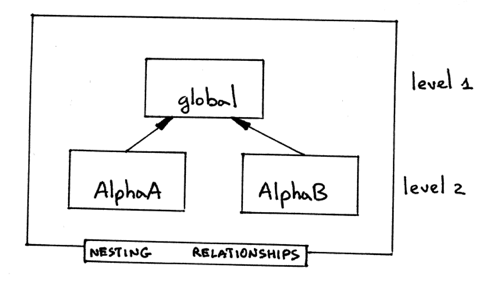``````\$ python scope03b.py
ENTER scope: global
Insert: INTEGER
Insert: REAL
Lookup: REAL
Insert: x
Lookup: REAL
Insert: y
Insert: AlphaA
ENTER scope: AlphaA
Insert: INTEGER
Insert: REAL
Lookup: INTEGER
Insert: a
Lookup: INTEGER
Insert: y

SCOPE (SCOPED SYMBOL TABLE)
===========================
Scope name     : AlphaA
Scope level    : 2
Enclosing scope: global
Scope (Scoped symbol table) contents
------------------------------------
INTEGER: <BuiltinTypeSymbol(name='INTEGER')>
REAL: <BuiltinTypeSymbol(name='REAL')>
a: <VarSymbol(name='a', type='INTEGER')>
y: <VarSymbol(name='y', type='INTEGER')>

LEAVE scope: AlphaA
Insert: AlphaB
ENTER scope: AlphaB
Insert: INTEGER
Insert: REAL
Lookup: INTEGER
Insert: a
Lookup: INTEGER
Insert: b

SCOPE (SCOPED SYMBOL TABLE)
===========================
Scope name     : AlphaB
Scope level    : 3
Enclosing scope: AlphaA
Scope (Scoped symbol table) contents
------------------------------------
INTEGER: <BuiltinTypeSymbol(name='INTEGER')>
REAL: <BuiltinTypeSymbol(name='REAL')>
a: <VarSymbol(name='a', type='INTEGER')>
b: <VarSymbol(name='b', type='INTEGER')>

LEAVE scope: AlphaB

SCOPE (SCOPED SYMBOL TABLE)
===========================
Scope name     : global
Scope level    : 1
Enclosing scope: None
Scope (Scoped symbol table) contents
------------------------------------
INTEGER: <BuiltinTypeSymbol(name='INTEGER')>
REAL: <BuiltinTypeSymbol(name='REAL')>
x: <VarSymbol(name='x', type='REAL')>
y: <VarSymbol(name='y', type='REAL')>
AlphaA: <ProcedureSymbol(name=AlphaA, parameters=[<VarSymbol(name='a', type='INTEGER')>])>

LEAVE scope: global
``````

1. 与在嵌套关系图所示的两个作用域的级别相反，我们有三个级别
2. 在全局作用域内的内容不包含 AlphaB 在里面，只有 AlphaA

1. 当 ENTER 进一个 ProgramProcedureDecl 节点，创建一个新的作用域，并将其分配给 self.current_scope
2. 当即将LEAVE ProgramProcedureDecl 节点，恢复 self.current_scope 的值。

1. 当你访问 ProgramProcedureDecl 节点，将一个新的作用域入栈并调整栈指针 self.current_scope 指向栈的顶部，就是最近进栈的作用域。
2. 当你要离开的节点，你从堆栈中弹出作用域，你还可以调整栈指针指向之前的作用域，这是现在的栈顶。

``````\$ python scope03c.py
ENTER scope: global
Insert: INTEGER
Insert: REAL
Lookup: REAL
Insert: x
Lookup: REAL
Insert: y
Insert: AlphaA
ENTER scope: AlphaA
Insert: INTEGER
Insert: REAL
Lookup: INTEGER
Insert: a
Lookup: INTEGER
Insert: y

SCOPE (SCOPED SYMBOL TABLE)
===========================
Scope name     : AlphaA
Scope level    : 2
Enclosing scope: global
Scope (Scoped symbol table) contents
------------------------------------
INTEGER: <BuiltinTypeSymbol(name='INTEGER')>
REAL: <BuiltinTypeSymbol(name='REAL')>
a: <VarSymbol(name='a', type='INTEGER')>
y: <VarSymbol(name='y', type='INTEGER')>

LEAVE scope: AlphaA
Insert: AlphaB
ENTER scope: AlphaB
Insert: INTEGER
Insert: REAL
Lookup: INTEGER
Insert: a
Lookup: INTEGER
Insert: b

SCOPE (SCOPED SYMBOL TABLE)
===========================
Scope name     : AlphaB
Scope level    : 2
Enclosing scope: global
Scope (Scoped symbol table) contents
------------------------------------
INTEGER: <BuiltinTypeSymbol(name='INTEGER')>
REAL: <BuiltinTypeSymbol(name='REAL')>
a: <VarSymbol(name='a', type='INTEGER')>
b: <VarSymbol(name='b', type='INTEGER')>

LEAVE scope: AlphaB

SCOPE (SCOPED SYMBOL TABLE)
===========================
Scope name     : global
Scope level    : 1
Enclosing scope: None
Scope (Scoped symbol table) contents
------------------------------------
INTEGER: <BuiltinTypeSymbol(name='INTEGER')>
REAL: <BuiltinTypeSymbol(name='REAL')>
x: <VarSymbol(name='x', type='REAL')>
y: <VarSymbol(name='y', type='REAL')>
AlphaA: <ProcedureSymbol(name=AlphaA, parameters=[<VarSymbol(name='a', type='INTEGER')>])>
AlphaB: <ProcedureSymbol(name=AlphaB, parameters=[<VarSymbol(name='a', type='INTEGER')>])>

LEAVE scope: global
``````#### 嵌套作用域和名称解析

``````program Main;
var x, y: real;

procedure Alpha(a : integer);
var y : integer;
begin
x := a + x + y;
end;

begin { Main }

end.  { Main }
``````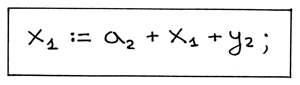1. 因为语义分析先访问赋值语句的右侧，我们从算术表达式 a + x + y 中变量引用 a 开始。我们开始在词法最接近的作用域搜索 a 声明，就是 Alpha 作用域。 Alpha 作用域包含 Alpha 过程中的变量声明，包括过程的形式参数。我们在 Alpha 作用域找到 a 的声明：它是 Alpha 过程的形式参数 a ——类型 integer 的变量符号。我们通常在解析名称时用眼睛扫描源代码进行搜索（记住，a2 不是变量的名称，2是这里的下标，变量名是 a ）：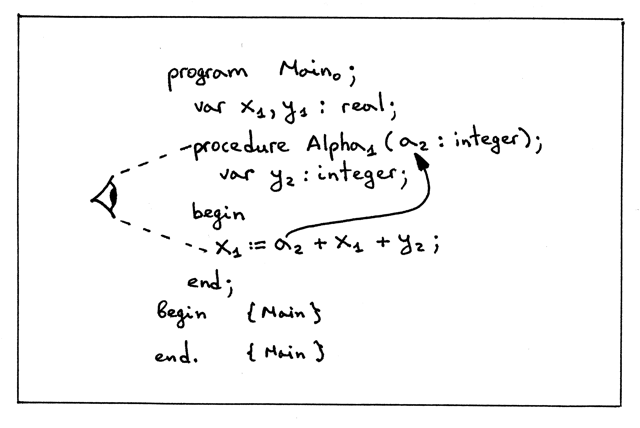2. 现在看下算术表达式 a + x + y. 中变量引用 x 。同样，我们首先在词法最接近作用域寻找 x 的声明。词法最接近的作用域是第2级的 Alpha 作用域。作用域包含了 Alpha 过程中的变量声明，包括过程的形式参数。我们在此作用域级别（在 Alpha 作用域）没有找到 x ，所以从链接上溯到全局作用域，并继续搜索。我们的搜索成功，因为全局作用域内有名称为 x 的变量符号：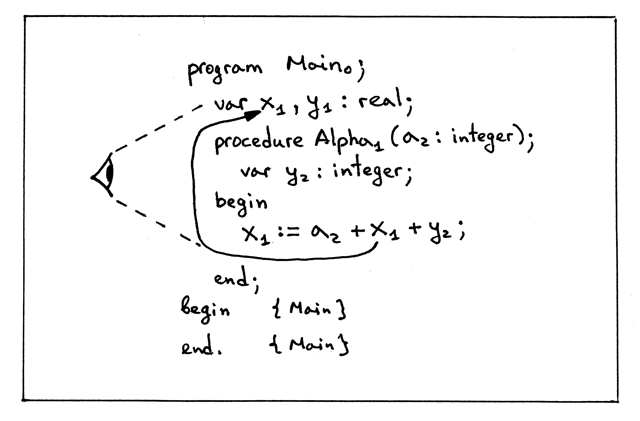3. 现在，让我们来看看算术表达式 a + x + y. 中变量引用 y 。我们在词法最接近作用域 Alpha 作用域上发现它的声明。在 Alpha 作用域变量 y 具有类型 integer （如果在 Alpha 作用域内没有声明 y ，我们将扫描文本，并在外层/全局作用域找到 y 而在这种情况它是 real 类型）：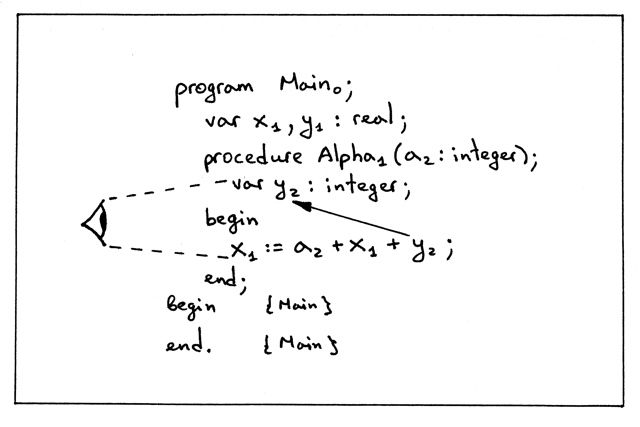4. 最后，赋值语句 a + x + y. 的左侧变量 x ，它和右侧算术表达式变量引用 x 解析为相同的声明：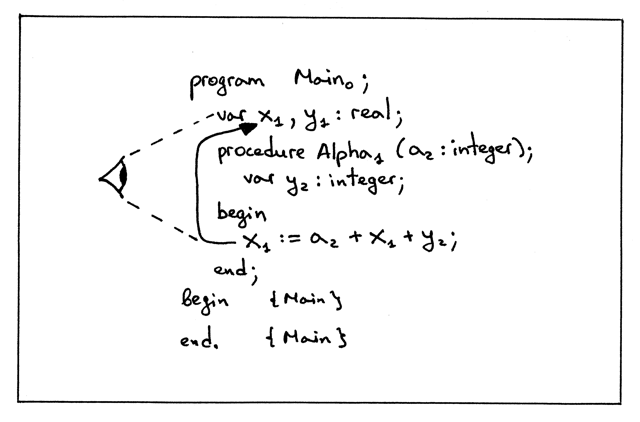``````def lookup(self, name):
print('Lookup: %s. (Scope name: %s)' % (name, self.scope_name))
# 'symbol' is either an instance of the Symbol class or None
symbol = self._symbols.get(name)

if symbol is not None:
return symbol

# recursively go up the chain and lookup the name
if self.enclosing_scope is not None:
return self.enclosing_scope.lookup(name)
``````

1. 在当前作用域内搜索符号的名字。如果找到符号，返回它。
2. 如果没有找到符号，递归遍历树并在作用域链上向上搜索符号。你不必做递归查询，可以把它改写成一个迭代的形式; 重要的部分是沿着嵌套作用域的链接到它的封闭作用域，在那搜索符号或上溯树直到符号被发现或没有遗留的作用域，因为你已经到达了作用域树的顶部。
3. lookup 方法也在括号中打印了作用域的名称，当不能在当前作用域内找到符号时，在查找发生时能更清晰地表明在链条中上溯搜索符号的行为。

``````\$ python scope04a.py
ENTER scope: global
Insert: INTEGER
Insert: REAL
Lookup: REAL. (Scope name: global)
Insert: x
Lookup: REAL. (Scope name: global)
Insert: y
Insert: Alpha
ENTER scope: Alpha
Lookup: INTEGER. (Scope name: Alpha)
Lookup: INTEGER. (Scope name: global)
Insert: a
Lookup: INTEGER. (Scope name: Alpha)
Lookup: INTEGER. (Scope name: global)
Insert: y
Lookup: a. (Scope name: Alpha)
Lookup: x. (Scope name: Alpha)
Lookup: x. (Scope name: global)
Lookup: y. (Scope name: Alpha)
Lookup: x. (Scope name: Alpha)
Lookup: x. (Scope name: global)

SCOPE (SCOPED SYMBOL TABLE)
===========================
Scope name     : Alpha
Scope level    : 2
Enclosing scope: global
Scope (Scoped symbol table) contents
------------------------------------
a: <VarSymbol(name='a', type='INTEGER')>
y: <VarSymbol(name='y', type='INTEGER')>

LEAVE scope: Alpha

SCOPE (SCOPED SYMBOL TABLE)
===========================
Scope name     : global
Scope level    : 1
Enclosing scope: None
Scope (Scoped symbol table) contents
------------------------------------
INTEGER: <BuiltinTypeSymbol(name='INTEGER')>
REAL: <BuiltinTypeSymbol(name='REAL')>
x: <VarSymbol(name='x', type='REAL')>
y: <VarSymbol(name='y', type='REAL')>
Alpha: <ProcedureSymbol(name=Alpha, parameters=[<VarSymbol(name='a', type='INTEGER')>])>

LEAVE scope: global
``````

1. 注意语义分析如何在插入变量符号 a 前查找 INTEGER 内置型符号。它首先在当前作用域 Alpha 搜索 INTEGER ，，没有找到，然后上溯树一路到全局作用域内并发现了符号有：

``````ENTER scope: Alpha
Lookup: INTEGER. (Scope name: Alpha)
Lookup: INTEGER. (Scope name: global)
Insert: a
``````
2. 还要注意分析器如何解析赋值语句 x := a + x + y 的变量引用：

``````Lookup: a. (Scope name: Alpha)
Lookup: x. (Scope name: Alpha)
Lookup: x. (Scope name: global)
Lookup: y. (Scope name: Alpha)
Lookup: x. (Scope name: Alpha)
Lookup: x. (Scope name: global)
``````

分析器在当前作用域内开始其搜索，然后上溯树一路到全局作用域。

``````program Main;
var x, y: real;

procedure Alpha(a : integer);
var y : integer;
begin
x := b + x + y; { ERROR here! }
end;

begin { Main }

end.  { Main }
``````

``````\$ python scope04b.py
ENTER scope: global
Insert: INTEGER
Insert: REAL
Lookup: REAL. (Scope name: global)
Insert: x
Lookup: REAL. (Scope name: global)
Insert: y
Insert: Alpha
ENTER scope: Alpha
Lookup: INTEGER. (Scope name: Alpha)
Lookup: INTEGER. (Scope name: global)
Insert: a
Lookup: INTEGER. (Scope name: Alpha)
Lookup: INTEGER. (Scope name: global)
Insert: y
Lookup: b. (Scope name: Alpha)
Lookup: b. (Scope name: global)
``````

#### 源到源编译器

``````program Main;
var x, y: real;

procedure Alpha(a : integer);
var y : integer;
begin
x := a + x + y;
end;

begin { Main }

end.  { Main }
``````

``````program Main0;
var x1 : REAL;
var y1 : REAL;
procedure Alpha1(a2 : INTEGER);
var y2 : INTEGER;

begin
<x1:REAL> := <a2:INTEGER> + <x1:REAL> + <y2:INTEGER>;
end; {END OF Alpha}

begin

end. {END OF Main}
``````

1. 每个声明应打印在单独的行，所以，如果我们在输入的Pascal 程序中有多个声明，编译后的输出应该每个声明有单独的一行。我们可以在上面看到，例如，行 var x, y : real; 怎么被转换成多行的。
2. 每个名字应该有下标对应于各自的作用域级别的数字。
3. 每个变量引用，在除了被加下标，也应输出为下面的形式：
4. 编译器也应该在每个块的末尾添加 {END OF … }形式的注释 ，这里的省略号将被程序名称或过程名称取代。这将帮助我们更快识别程序的文本边界。

``````program Main;
var x, y : real;
var z : integer;

procedure AlphaA(a : integer);
var y : integer;
begin { AlphaA }
x := a + x + y;
end;  { AlphaA }

procedure AlphaB(a : integer);
var b : integer;
begin { AlphaB }
end;  { AlphaB }

begin { Main }
end.  { Main }
``````

``````\$ python src2srccompiler.py nestedscopes03.pas
program Main0;
var x1 : REAL;
var y1 : REAL;
var z1 : INTEGER;
procedure AlphaA1(a2 : INTEGER);
var y2 : INTEGER;

begin
<x1:REAL> := <a2:INTEGER> + <x1:REAL> + <y2:INTEGER>;
end; {END OF AlphaA}
procedure AlphaB1(a2 : INTEGER);
var b2 : INTEGER;

begin

end; {END OF AlphaB}

begin

end. {END OF Main}
``````

``````program Main;
var b, x, y : real;
var z : integer;

procedure AlphaA(a : integer);
var b : integer;

procedure Beta(c : integer);
var y : integer;

procedure Gamma(c : integer);
var x : integer;
begin { Gamma }
x := a + b + c + x + y + z;
end;  { Gamma }

begin { Beta }

end;  { Beta }

begin { AlphaA }

end;  { AlphaA }

procedure AlphaB(a : integer);
var c : real;
begin { AlphaB }
c := a + b;
end;  { AlphaB }

begin { Main }
end.  { Main }
``````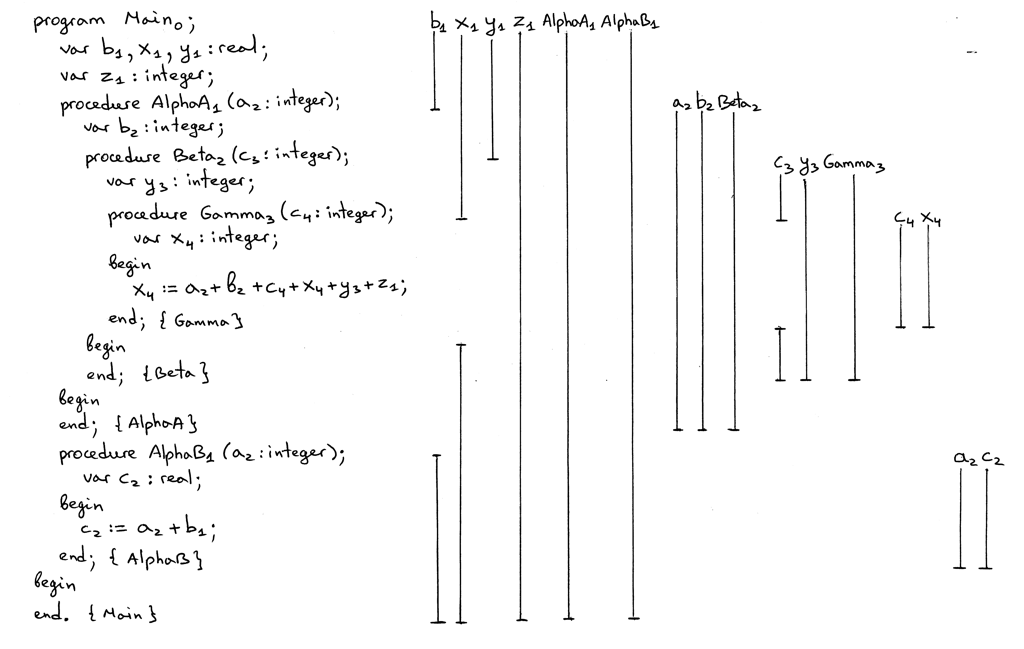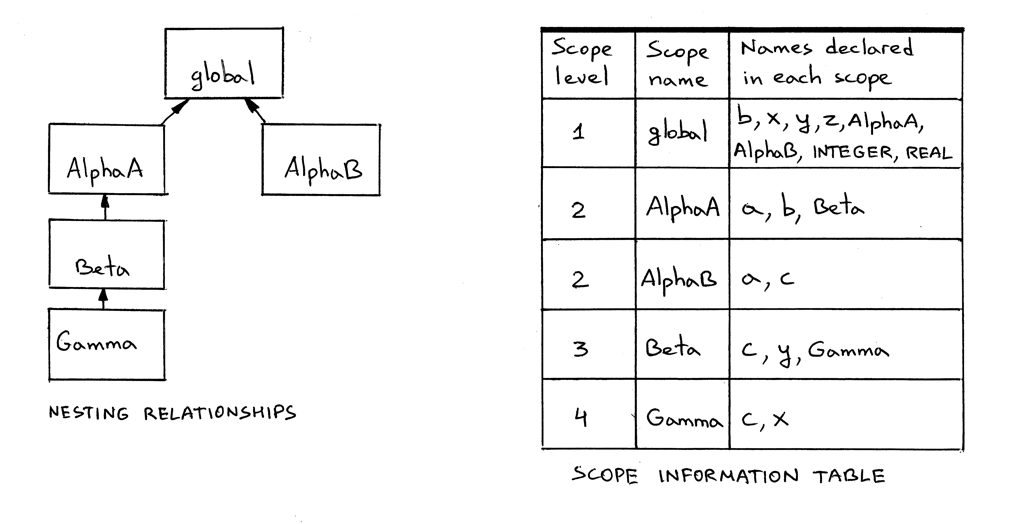``````\$ python src2srccompiler.py nestedscopes04.pas
program Main0;
var b1 : REAL;
var x1 : REAL;
var y1 : REAL;
var z1 : INTEGER;
procedure AlphaA1(a2 : INTEGER);
var b2 : INTEGER;
procedure Beta2(c3 : INTEGER);
var y3 : INTEGER;
procedure Gamma3(c4 : INTEGER);
var x4 : INTEGER;

begin
<x4:INTEGER> := <a2:INTEGER> + <b2:INTEGER> + <c4:INTEGER> + <x4:INTEGER> + <y3:INTEGER> + <z1:INTEGER>;
end; {END OF Gamma}

begin

end; {END OF Beta}

begin

end; {END OF AlphaA}
procedure AlphaB1(a2 : INTEGER);
var c2 : REAL;

begin
<c2:REAL> := <a2:INTEGER> + <b1:REAL>;
end; {END OF AlphaB}

begin

end. {END OF Main}
``````

• 垂直线条画的路线显示的声明的作用域。
• 在一个作用域的洞表示变量在嵌套作用域重新声明。
• AlphaAAlphaB 是在全局作用域内声明。
• AlphaAAlphaB 的声明引入新的作用域。
• 作用域如何相互嵌套，以及嵌套的关系。
• 为什么不同的名称，包括在赋值语句中的变量引用的下标会是这样的。换句话说，名称解析和链式作用域符号表上的特殊 lookup 方法是的工作如何的。

``````\$ python scope05.py nestedscopes04.pas
``````

``````def lookup(self, name, current_scope_only=False):
print('Lookup: %s. (Scope name: %s)' % (name, self.scope_name))
# 'symbol' is either an instance of the Symbol class or None
symbol = self._symbols.get(name)

if symbol is not None:
return symbol

if current_scope_only:
return None

# recursively go up the chain and lookup the name
if self.enclosing_scope is not None:
return self.enclosing_scope.lookup(name)
``````

``````def visit_VarDecl(self, node):
type_name = node.type_node.value
type_symbol = self.current_scope.lookup(type_name)

# We have all the information we need to create a variable symbol.
# Create the symbol and insert it into the symbol table.
var_name = node.var_node.value
var_symbol = VarSymbol(var_name, type_symbol)

# Signal an error if the table alrady has a symbol
# with the same name
if self.current_scope.lookup(var_name, current_scope_only=True):
raise Exception(
"Error: Duplicate identifier '%s' found" % var_name
)

self.current_scope.insert(var_symbol)
``````

``````\$ python scope05.py nestedscopes02.pas
ENTER scope: global
Insert: INTEGER
Insert: REAL
Lookup: REAL. (Scope name: global)
Lookup: x. (Scope name: global)
Insert: x
Lookup: REAL. (Scope name: global)
Lookup: y. (Scope name: global)
Insert: y
Insert: Alpha
ENTER scope: Alpha
Lookup: INTEGER. (Scope name: Alpha)
Lookup: INTEGER. (Scope name: global)
Insert: a
Lookup: INTEGER. (Scope name: Alpha)
Lookup: INTEGER. (Scope name: global)
Lookup: y. (Scope name: Alpha)
Insert: y
Lookup: a. (Scope name: Alpha)
Lookup: x. (Scope name: Alpha)
Lookup: x. (Scope name: global)
Lookup: y. (Scope name: Alpha)
Lookup: x. (Scope name: Alpha)
Lookup: x. (Scope name: global)

SCOPE (SCOPED SYMBOL TABLE)
===========================
Scope name     : Alpha
Scope level    : 2
Enclosing scope: global
Scope (Scoped symbol table) contents
------------------------------------
a: <VarSymbol(name='a', type='INTEGER')>
y: <VarSymbol(name='y', type='INTEGER')>

LEAVE scope: Alpha

SCOPE (SCOPED SYMBOL TABLE)
===========================
Scope name     : global
Scope level    : 1
Enclosing scope: None
Scope (Scoped symbol table) contents
------------------------------------
INTEGER: <BuiltinTypeSymbol(name='INTEGER')>
REAL: <BuiltinTypeSymbol(name='REAL')>
x: <VarSymbol(name='x', type='REAL')>
y: <VarSymbol(name='y', type='REAL')>
Alpha: <ProcedureSymbol(name=Alpha, parameters=[<VarSymbol(name='a', type='INTEGER')>])>

LEAVE scope: global
``````

``````program Main;
var x, y: real;

procedure Alpha(a : integer);
var y : integer;
var a : real;  { ERROR here! }
begin
x := a + x + y;
end;

begin { Main }

end.  { Main }
``````

``````\$ python scope05.py dupiderror.pas
ENTER scope: global
Insert: INTEGER
Insert: REAL
Lookup: REAL. (Scope name: global)
Lookup: x. (Scope name: global)
Insert: x
Lookup: REAL. (Scope name: global)
Lookup: y. (Scope name: global)
Insert: y
Insert: Alpha
ENTER scope: Alpha
Lookup: INTEGER. (Scope name: Alpha)
Lookup: INTEGER. (Scope name: global)
Insert: a
Lookup: INTEGER. (Scope name: Alpha)
Lookup: INTEGER. (Scope name: global)
Lookup: y. (Scope name: Alpha)
Insert: y
Lookup: REAL. (Scope name: Alpha)
Lookup: REAL. (Scope name: global)
Lookup: a. (Scope name: Alpha)
Error: Duplicate identifier 'a' found
``````

#### 总结

• 我们学习了作用域，为什么它很有用，以及如何在代码中实现它。
• 我们学习了嵌套作用域和链式作用域符号表如何被用来实现嵌套作用域。
• 我们学会了如何编写一个语义分析器：遍历AST，建立作用域符号表，把它们链在一起，并执行各种语义检查。
• 我们学习了名称解析和语义分析如何使用它们的链式作用域符号表（作用域）解析名称到声明， 以及 lookup 方法如何递归的在作用域树上溯链接以找到对应于特定名称的声明。
• 我们学习到在语义分析器遍历AST时构建作用域树，当 ENTER 某些AST节点时，在作用域符号表栈顶入栈一个新的作用域，当 LEAVing 节点时，在从栈顶中取出作用域，使得作用域树就像作用域符号表栈的集合。
• 我们学习了如何编写一个源到源编译器，它对学习嵌套作用域、作用域级别和名称解析是一个非常有用的工具。

#### 练习1. 你已经看到了整个文章图片中程序声明 Main 名称的下标为零。我还提到，程序的名字是不在全局作用域内的，它是在具有零级别的其他一些外部作用域中。扩展spi.py并创建在0级别的新作用域 builtins ，，并移动内置类型 INTEGER 和 REAL 到该作用域。为了好玩和实践，还可以更新代码把程序名字放入该作用域内。
2. 对于在nestedscopes04.pas中源程序做以下几点：
1. 在一张纸上写下源Pascal程序
2. 标在程序中对每个名称做下表表明名称解析到的声明的作用域级别。
3. 为每个名称声明（变量和过程）画垂直线，以在视觉上显示出其作用域。不要在绘画时忘了作用域空洞和他们的意思。
4. 为程序写一个源到源编译器而不看这篇文章中的示例源到源编译器。
5. 使用原始src2srccompiler.py程序来验证从你的编译器的输出，你是否在练习（2.2）正确对名称做下标。
3. 修改源到源编译器为内置类型INTEGER和REAL添加下标
4. 取消以下块在spi.py中的注释

``````# interpreter = Interpreter(tree)
# result = interpreter.interpret()
# print('')
# print('Run-time GLOBAL_MEMORY contents:')
# for k, v in sorted(interpreter.GLOBAL_MEMORY.items()):
#     print('%s = %s' % (k, v))
``````

part10.pas文件作为输入运行解释器：

``````\$ python spi.py part10.pas
``````

确认问题并将缺失的方法添加到语义分析器中。